Technical Article

# The Importance of Complex Conjugates

November 17, 2016 by Sneha H.L.

## This article provides insight into the importance of complex conjugates in electrical engineering.

This article provides insight into the importance of complex conjugates in electrical engineering.

### Complex Numbers

Complex numbers are numbers which are represented in the form $$z = x + i y$$, where x and y are the real and imaginary parts (respectively) and $$i =\sqrt{-1}$$.

Complex numbers can also be represented in polar form, which has a magnitude term and an angular term. The magnitude of a complex number $$z = x + i y$$ is given as $$|z| = \sqrt{x^{2}+y^{2}}$$, and its angular term is calculated as $$\theta = tan^{-1}\left(\frac{y}{x}\right)$$.

A complex number can be represented graphically as a point in two-dimensional space, with the real and imaginary parts corresponding to the x- and y-axes, respectively, as seen in Figure 1(a).

A complex number can also be represented in polar form, which consists of a vector of length |z| originating from the origin and pointing outwards at an angle equal to θ, as seen in Figure 1(b).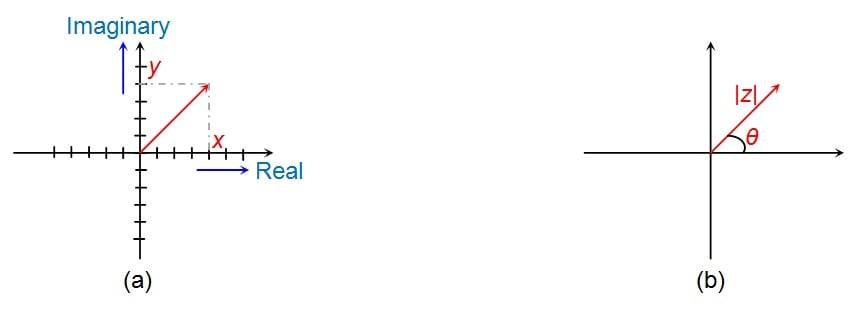### Complex Conjugates

If $$z = x + i y$$ is a complex number, then the complex conjugate, denoted by $$\overline{z}$$ or $${z^{*}}$$, is $$x - i y$$. Thus, we find the complex conjugate simply by changing the sign of the imaginary part (the real part does not change).

If the complex number is expressed in polar form, we obtain the complex conjugate by changing the sign of the angle (the magnitude does not change).

Figure 2(a) and 2(b) are, respectively, Cartesian-form and polar-form representations of the complex conjugate.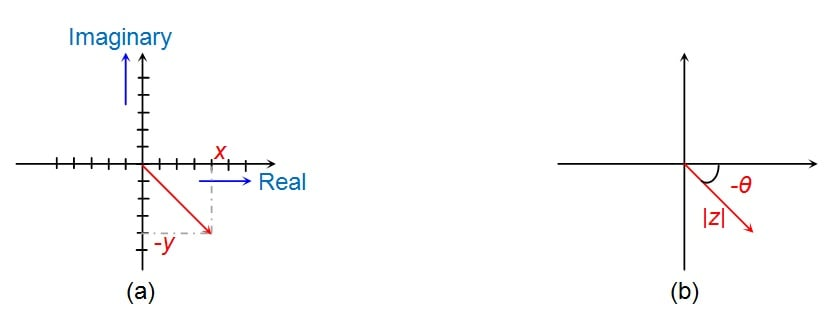##### Figure 2: Complex conjugate representation in (a) Cartesian form and (b) polar form. Keep in mind that Figure 2(a) and 2(b) are two different ways of describing the same point.

Let's take a closer look at these figures. By comparing Figures 1(a) and 2(a), we can see that the span along the real axis is the same, whereas the span along the imaginary axis is equal in length but opposite in direction. Thus, we can say that the complex conjugate of any complex number corresponds to a reflection across the real axis.

This is evident also in the polar-form graphs: the length of the vector is the same and the angle's magnitude has not changed, but the orientation relative to the real axis is different because the angle now has the opposite sign.

Now let's consider a complex vector that is rotating around the origin at an angular frequency ɷ. (One complete rotation corresponds to 2π radians, and thus one cycle per second is equivalent to an angular frequency of 2π radians per second.) In this case, the complex vector is referred to as a phasor, and phasors can represent sinusoidal variations. Thus, complex numbers can be used to model systems involving sinusoidal phenomena such as physical waves and AC electrical signals.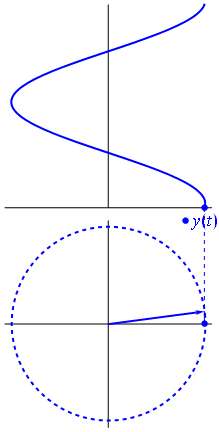### The Importance of Complex Conjugates

Now, let's consider some different contexts in which complex conjugates are useful.

#### Case I: Vector Rotation

The angle of a vector can be rotated via complex multiplication. For example, multiplying complex vector z by the complex vector $$1 + i$$ will rotate z by 45°. To reverse this rotation, we multiply by the complex conjugate of $$1 + i$$, namely, $$1 - i$$.

#### Case II: Expression Simplification

Complex conjugates are helpful when one needs to simplify expressions such as $$\frac{\left(3 + 4i\right)}{\left(-5 + 6i\right)}$$. This is because, when we multiply the numerator and denominator of such an expression by the complex conjugate of the denominator, we get a single complex number.

Consider this example:

$$\frac{\left(3 + 4i\right)}{\left(-5 + 6i\right)} \times \frac{\left(-5 - 6i\right)}{\left(-5 - 6i\right)} = \frac{-15 - 20i - 18 i- 24 i^{2}}{\left(-5\right)^{2} - \left(6i\right)^{2}}$$

$$=\frac{-15 - 38 i+ 24}{25 - 36 \left(i\right)^{2}}$$

$$= \frac{9 - 38 i}{25 + 36}$$

$$= \frac{9 - 38 i}{61} = \frac{9}{61} - \frac{38}{61} i$$

This kind of simplification is referred to as rationalization. The result has fractions for its real and imaginary parts, but because the imaginary unit does not appear in the denominator, the simplified expression is more mathematically standard and more convenient to work with than the original expression.

#### Case III: Finding Roots of Polynomial Expressions

Next, suppose that we need to find the roots of a polynomial expression. To simplify this process, we can turn to an axiom of mathematics which states that complex roots always appear in pairs. Thus, if we find that the root of a particular equation is $$x + iy$$, then its complex conjugate $$x - iy$$ is also a root.

#### Case IV: Calculating DFTs and IDFTs

Conjugate pairs are also involved when we need to find a Discrete Fourier Transform (DFT) or Inverse Discrete Fourier Transform (IDFT). This is because the DFT and IDFT of real sequences exhibit conjugate symmetry about the midpoint.

Figure 3 shows an example of a 5-point DFT of a real sequence that exhibits conjugate symmetry with respect to points $$P_1$$ and $$P_2$$. That is, points $$P_1^*$$ and $$P_2^*$$ are the complex conjugate pairs of points $$P_1$$ and $$P_2$$, respectively.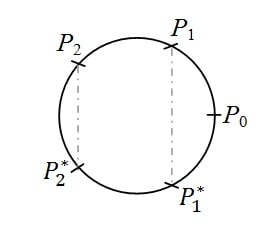##### Figure 3: Representation of conjugate symmetry exhibited by DFTs and IDFTs

For example, let us assume that $$(1, 0.25 + 0.3i, 0.5 - 0.2i, 0.3 - 0.6i)$$ are the first four points of the 7-point DFT of a real sequence. Then, the remaining three points will have to be $$(0.25 - 0.3i, 0.5 + 0.2i, 0.3 + 0.6i)$$.

This is very useful as it reduces the computational burden almost to half, and the improvement becomes more pronounced as the length of the DFT/IDFT increases.

#### Case V: AC Circuit Analysis

Here we have an electrical network consisting of resistors, an inductor, and a capacitor in addition to a current source and a voltage source.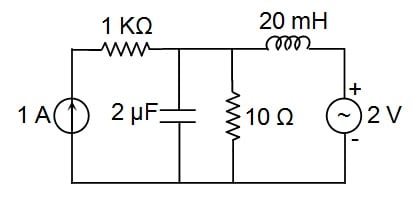##### Figure 4: An AC electrical network

Note: For this example we will use j instead of i for the imaginary unit. The letter j is preferred in the context of electrical engineering because it eliminates the possibility of confusion between the imaginary unit and the letter used to denote electric current.

The operation of circuits that include capacitors or inductors is less straightforward than resistor-only circuits because capacitors and inductors introduce time dependency into the behavior of a circuit. Such circuits can be analyzed in the time domain, but often the necessary information is more readily obtained by working in the frequency domain, which requires that we assign an impedance to inductors and capacitors. The impedance of an inductor with inductance L is jωL, and the impedance of a capacitor with capacitance C is 1/(jωC).

Because the circuit elements now involve complex numbers, mathematical analysis can lead to expressions that require rationalization via the complex conjugate. In addition, we have the Maximum Power Transfer Theorem, which states that a given circuit will transfer maximum power to a load when the impedance of the load is the complex conjugate of the circuit's Thévenin impedance.

#### Case VI: Probability

Next, let us consider the issue of probability. With ordinary physical phenomena, the probability of an event is the summation of the probabilities of all the ways in which the event can occur. Similarly, the probability of something in the case of quantum physics will be the square of the absolute value obtained by summing up the probability amplitudes of all the different ways in which it can occur.

Here, it is important to note that the probability amplitude, which describes the behavior of the system in terms of the relationship between the system's wave function and the results of observations performed for that system, is a complex number. Thus, in order to obtain the probability as a real value, we will be forced to use the complex conjugate.

### Conclusion

Having discussed all these cases, we can conclude that the complex conjugate facilitates the analysis and design of systems—including electrical systems—that are modeled using complex numbers.

• Share• L
LEhenson November 25, 2016

Great article, however start with acknowledging the 1800’s “building block: for the above article, with understanding: Fourier Transform! SEE: https://en.wikipedia.org/wiki/Fourier_transform

Like.
•snehahl November 27, 2016
Thank you.
Like.
• A
Arthur Anderson November 28, 2016

As an electrical engineer, I’d like to point out that we do not use I as the unity imaginary number.  Instead we use j as I is ALWAYS a current.  Also, it is good to point out why things come in complex conjugate pairs.  The reason is simple, we live in the real world.  If the pairs were not complex conjugates, then there would be a complex coefficient in the differential equation describing the system.

Like.
•LymanT March 24, 2017

Great article. I was just reviewing complex numbers and how they pertain to different AC analysis.

Like.
•snehahl March 24, 2017
Thank you.
Like.
•Lonne Mays March 25, 2017

Thumbs up!  A great article providing both an overview and review of the subject.

Like.
•snehahl March 27, 2017
Thank you for the compliments.
Like.